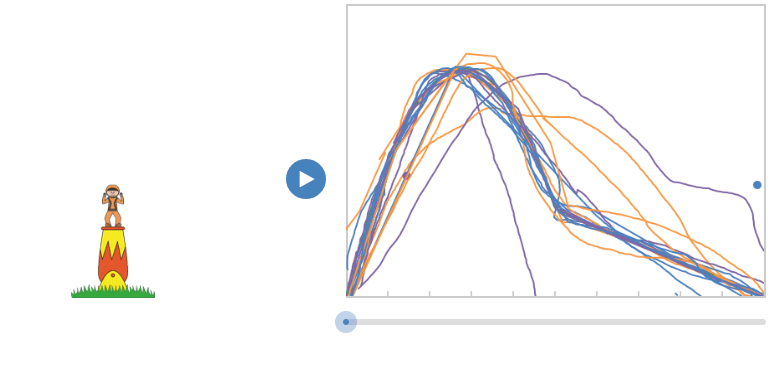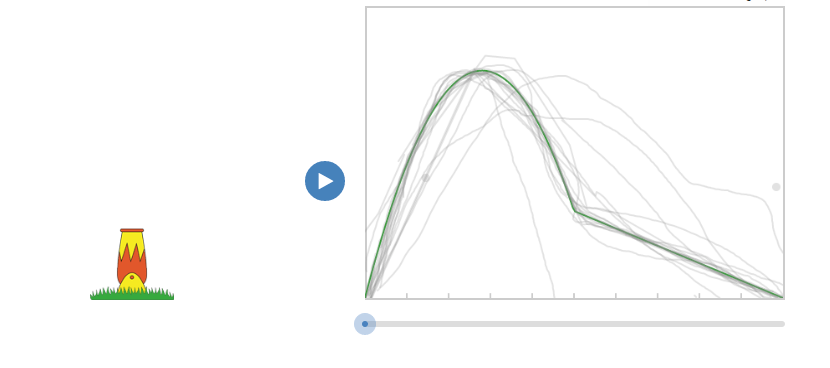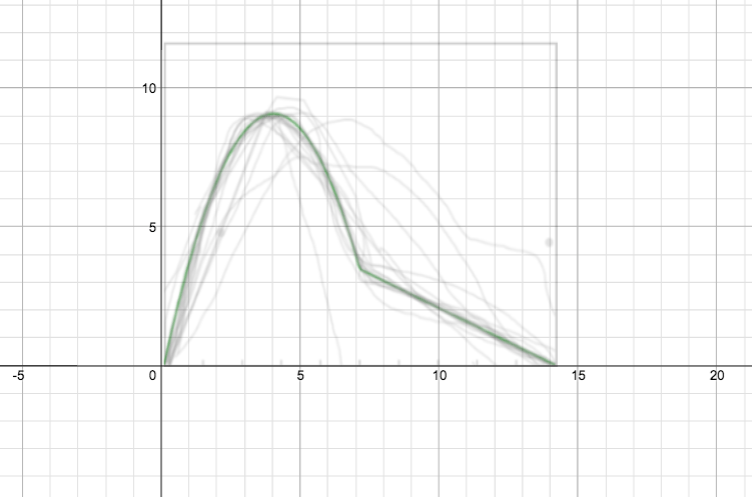# The Need for Speed!

Here was our lesson today on Instantaneous rates of change/slope of a tangent line

Yesterday we completed the awesome Desmos activity Function Carnival!! Some students were extremely competitive when trying to match the motion of the cannon shot to their graph…..some students not so much……here are their graphsAfter showing the kids this picture we talked about the motion of the guy and how it relates to the graph…..Questions we had

1. When would he be travelling the fastest? How do we know?
2. When would he stop? How do we know?
3. What happened after the parachute opened?

My goal here was to get talking about the speed of the guy. I asked:

#### If the guy was carrying a speedometer or a GPS…..what would his speed read out the moment before his parachute opened?

We stopped and made some estimates based on our intuition.

We talked about how to determine instantaneous speed from our knowledge of average rates of change, average speed, and slopes of secants lines.

We finally came to the conclusion that we could calculate the average rate of change between two points REALLY close together!!!!!! BINGO.

So I said let’s drop this image in Desmos! (we came to a decision that the cannon ball guy’s max height could have been around 9 metres).We looked at the graph and decided that it was HARD to estimate two points really close together.

So we added in some transformations to a basic quadratic function and fit the curve to the graph.I had pre-loaded some “extra” info in the sketch to demonstrate graphically the idea we were coming up with……the idea of looking a the slope of a secant line and as one point gets closer to the next the slope of the secant line approaches the slope of the tangent line.After looking at it graphically…..we used the equation we came up with to algebraically calculate the slope of the tangent line (the instantaneous rate of change).

We then practice that calculation using other points the students were interested in knowing about.

That was our lesson on Instantaneous Rates! Thanks Desmos!!## CONTACT ME